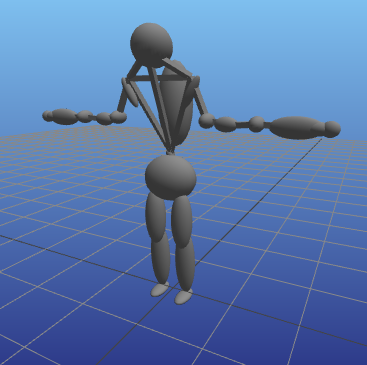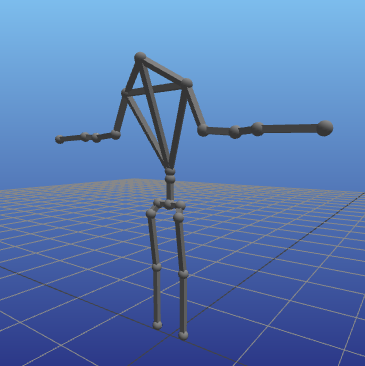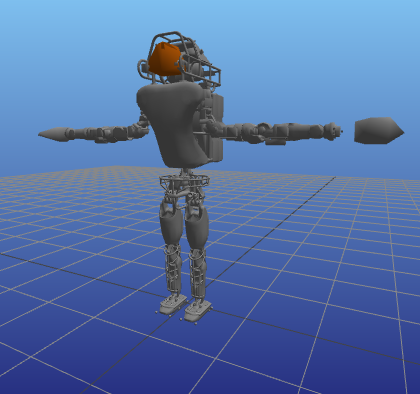dummy-link

10

1

5

6

# MechanismGeometries

This package implements several methods of generating or loading geometries associated with a RigidBodyDynamics.jl `Mechanism` in Julia. It is currently used by MeshCatMechanisms.jl but can also be used independently.

# Interface

This package exports one primary method:

``````visual_elements(mechanism::Mechanism, source::AbstractGeometrySource)
``````

`visual_elements` returns a vector of `VisualElement` structs, each of which contains:

• `frame`: A `CartesianFrame3D` indicating where the geometry is attached in the mechanism
• `geometry`: One of the GeometryBasics.jl types
• `color`: an RGBA color from ColorTypes.jl
• `transform`: a `Transformation` from CoordinateTransformations.jl indicating the pose of the geometry w.r.t its attached frame.

# Currently implemented sources

These demonstrations use the Boston Dynamics Atlas robot from AtlasRobot.jl.

``````using AtlasRobot
using MechanismGeometries
mechanism = AtlasRobot.mechanism()
``````

## Skeleton

``````Skeleton <: AbstractGeometrySource
``````

The `Skeleton` type uses only the joints and bodies in the mechanism itself to construct a visual representation of the robot's links. The sticks connect joints in the mechanism and the ellipsoids represent the mass and moment of inertia of each body:

``````visual_elements(mechanism, Skeleton())
``````The moment of inertia ellipsoids can also be turned off, leaving just the joint connections:

``````visual_elements(mechanism, Skeleton(inertias=false))
``````## URDF Visuals

``````URDFVisuals <: AbstractGeometrySource
``````

The `URDFVisuals` type loads the visual elements from a given URDF file (passed as either a filename or a parsed `XMLDocument` from LightXML.jl). One particularly useful argument is `package_path`, which accepts a list of strings to use as potential directories to search when encountering mesh files using the ROS `package://` syntax.

``````visual_elements(mechanism,
URDFVisuals(AtlasRobot.urdfpath(),
package_path=[AtlasRobot.packagepath()]))
``````### URDF Extensions

The following extensions to the URDF spec are parsed by MechanismGeometries.jl:

• `<plane normal="0 0 1"/>`: Represents an infinite plane perpendicular to the `normal` given as an x y z unit vector. Returns a MechanismGeometries.HyperPlane

03/07/2018

2 months ago

81 commits